# TranslationsPage 1

#### WATCH ALL SLIDES

Slide 1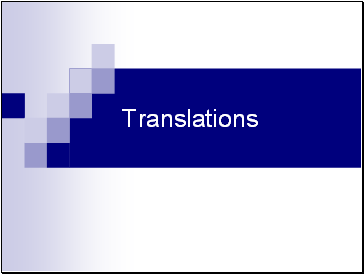## Translations

Slide 2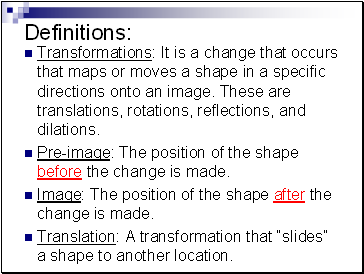Definitions:

Transformations: It is a change that occurs that maps or moves a shape in a specific directions onto an image. These are translations, rotations, reflections, and dilations.

Pre-image: The position of the shape before the change is made.

Image: The position of the shape after the change is made.

Translation: A transformation that “slides” a shape to another location.

Slide 3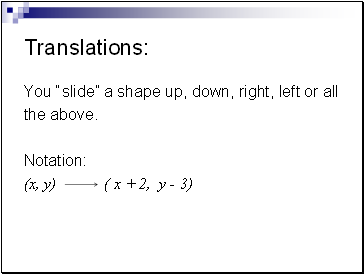Translations:

You “slide” a shape up, down, right, left or all

the above.

Notation:

(x, y) ( x + 2, y - 3)

Slide 4x

y

Pre-image

A (-2, 4)

B (-3, 2)

C (-1, 1)

Image

A’ (3, 4)

B’ (2, 2)

C’ (4, 1)

Transformation

(x, y) (x + 5, y + 0)

A

B

C

A’

B’

C’

Slide 5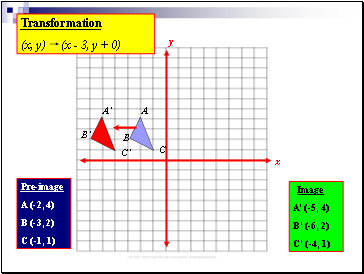x

y

Pre-image

A (-2, 4)

B (-3, 2)

C (-1, 1)

Image

A’ (-5, 4)

B’ (-6, 2)

C’ (-4, 1)

Transformation

(x, y) (x - 3, y + 0)

A

B

C

A’

B’

C’

Slide 6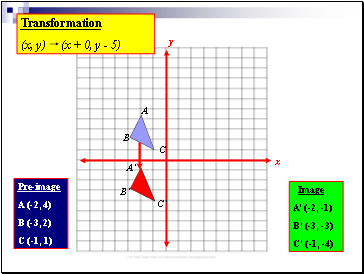x

y

Image

A’ (-2, -1)

B’ (-3, -3)

C’ (-1, -4)

Transformation

(x, y) (x + 0, y - 5)

A

B

C

A’

B’

C’

Pre-image

A (-2, 4)

B (-3, 2)

C (-1, 1)

Slide 7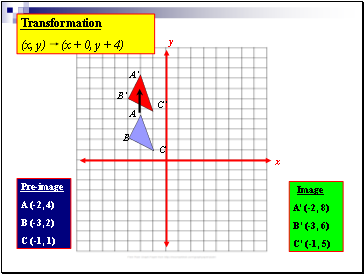x

y

Pre-image

A (-2, 4)

B (-3, 2)

C (-1, 1)

Image

A’ (-2, 8)

B’ (-3, 6)

C’ (-1, 5)

Transformation

(x, y) (x + 0, y + 4)

A

B

C

A’

B’

C’

Slide 8x

y

Pre-image

A (-2, 4)

B (-3, 2)

C (-1, 1)

Image

A’ (1, 0)

B’ (0, -2)

C’ (2, -3)

Transformation

(x, y) (x + 3, y - 4)

A

B

C

A’

B’

C’

Slide 9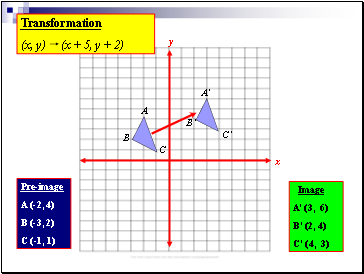x

y

Pre-image

A (-2, 4)

B (-3, 2)

C (-1, 1)

Image

A’ (3, 6)

B’ (2, 4)

C’ (4, 3)

Transformation

(x, y) (x + 5, y + 2)

A

B

C

A’

B’

C’

Slide 10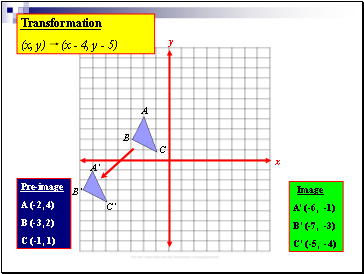x

y

Pre-image

A (-2, 4)

B (-3, 2)

C (-1, 1)

Image

A’ (-6, -1)

B’ (-7, -3)

C’ (-5, -4)

Transformation

(x, y) (x - 4, y - 5)

A

Go to page:
1  2